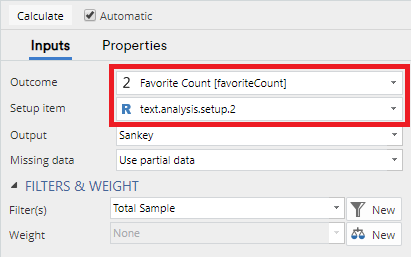# Text Analysis - Advanced - Predictive Tree

Related Videos

Chapter within Text Analysis in Q5 (Video)

Create a predictive tree which shows how an outcome variable is predicted by the results of a Text Analysis

This item can be used to create a predictive tree which shows how an outcome variable is predicted by the results of a text analysis. This means that the tree will describe which words and phrases from the text play a significant role in determining the value of the outcome. The text analysis must be done first, using Text Analysis - Setup Text Analysis, and all settings regarding the processing of the text are achieved by this setup item.

See this blog post for an example of predicting engagement from tweets.

## Example

In Displayr, go to Anything > Advanced Analysis > Text Analysis > Advanced > Predictive Tree.

In Q, go to Create > Text Analysis > Advanced > Predictive Tree

1. Under Inputs > Outcome select a Numeric, Categorical, or Ordered Categorical variable containing the data that is to be predicted.
2. Under Inputs > Setup item select a Text Analysis - Advanced - Setup Text Analysis object.
3. Change any other settings according to your requirements.
4. Ensure the Automatic box is checked, or click CalculateThe output generated will be a predictive tree:

## Options

Outcome A Numeric, Categorical, or Ordered Categorical variable containing the data that is to be predicted.

Setup item A text analysis item created by Text Analysis - Setup Text Analysis.

Output:

• Sankey: A Sankey Tree, which is a graphical representation of the tree which provides information about the sample contained in each of the tree branches.
• Tree: A more traditional, but plain-looking, tree diagram.
• Text: Textual information which contains the details of how the tree is split at each branch.
• Table: A table which displays the outcome variable next to the original text from the text analysis, and the transformed text from the text analysis. This allows you to see the outcomes that were given for each text response.

Missing data See Missing Data Options.

Pruning The type of post-pruning applied to the tree. Choices are:

• Minimum error: Prune back leaf nodes to create the tree with the smallest cross validation error.
• Smallest tree: Prune to create the smallest tree with cross validation error at most 1 standard error greater than the minimum error.
• None: Retain the tree as it has been built. Note that choosing this option without Early stopping is prone to overfitting.

Early stopping Whether to stop splitting nodes before the fit stops improving. Setting this may decrease the time to build the tree, potentially at the cost of not finding the tree with the best accuracy. See here for more detail.

Outcome category labels Whether to shorten category labels from categorical outcome variables. The choices are:

• Full labels: The complete labels.
• Abbreviated labels: Labels that have been shortened by taking the first few letters from each word.
• Letters: Letters from the alphabet where "a" corresponds to the first category, "b" to the second category, and so on.

Allow long-running calculations Predictors with m categories require evaluation of 2^(m - 1) split points. This may cause calculations to run for a long time. Checking this box allows categorical variables with more than 30 categories to be included in Predictors.

## Acknowledgements

See Machine Learning - Classification And Regression Trees (CART) for details on the trees that are used here.

## Code

var heading_text = "Predictive tree";
if (!!form.setObjectInspectorTitle)
else
form.dropBox({label: "Outcome",
types:["Variable: Numeric, Categorical, OrderedCategorical"],
name: "formOutcomeVariable",
prompt: "Variable to be predicted based on the text"});

form.dropBox({name: "formInput",
label: "Setup item",
types: ["R:wordBag"],
prompt: "An object from Text Analysis > Setup"});

form.comboBox({label: "Output",
alternatives: ["Sankey", "Tree", "Text", "Table"],
name: "formOutput",
default_value: "Sankey"});

form.comboBox({label: "Missing data",
alternatives: ["Error if missing data", "Exclude cases with missing data", "Use partial data", "Imputation (replace missing values with estimates)"],
name: "formMissing",
default_value: "Use partial data"});
form.comboBox({label: "Pruning", alternatives: ["Minimum error", "Smallest tree", "None"],
name: "formPruning", default_value: "Minimum error",
prompt: "Remove nodes after tree has been built"})
form.checkBox({label: "Early stopping", name: "formStopping", default_value: false,
prompt: "Stop building tree when fit does not improve"});
form.comboBox({label: "Outcome category labels", alternatives: ["Full labels", "Abbreviated labels", "Letters"],
name: "formOutcomeCategoryLabels", default_value: "Full labels",
prompt: "Labelling of outcome categories in the tree (not used if outcome is numeric)"})
form.checkBox({label: "Allow long-running calculations", name: "formLongRunningCalculations", default_value: false,
prompt: "Allow predictors with more than 30 categories"});

library(flipTextAnalysis)
library(flipTrees)
library(flipFormat)

# Create the word bag on which the term document matrix and subsequent analysis is based
tas <- formInput
tdm <- AsTermMatrix(tas, min.frequency = 1, subset = tas$subset) colnames(tdm) <- make.names(colnames(tdm)) my.data <- data.frame(QDataFrame(formOutcomeVariable), tdm) CheckDataForTextTree(my.data, weights = QPopulationWeight, subset = QFilter, missing = formMissing) pt.formula <- as.formula(paste(colnames(my.data), " ~ ", paste(colnames(tdm), collapse = " + "))) pt <- CART(pt.formula, data = my.data, subset = QFilter, weights = QPopulationWeight, output = formOutput, prune = formPruning, early.stopping = formStopping, outcome.level.treatment = formOutcomeCategoryLabels, long.running.calculations = formLongRunningCalculations) transformed.text <- if (!is.null(tas$transformed.text)) tas$transformed.text else CreateTransformedText(tas) # Combine Tree with text text.predictive.tree <- CreateTextTree(pt, outcome.variable = formOutcomeVariable, original.text = tas$original.text,
transformed.text = transformed.text,
output = formOutput)